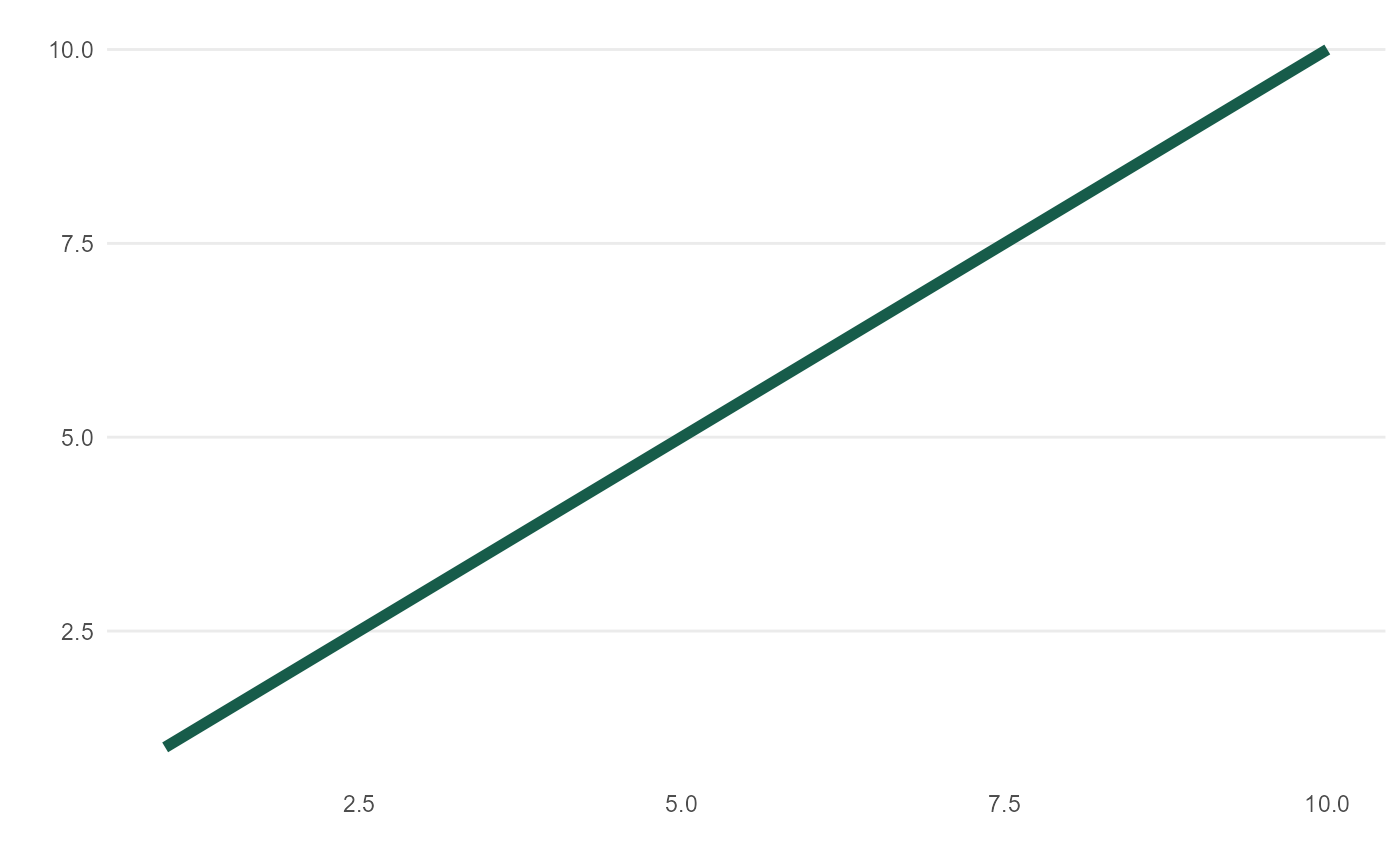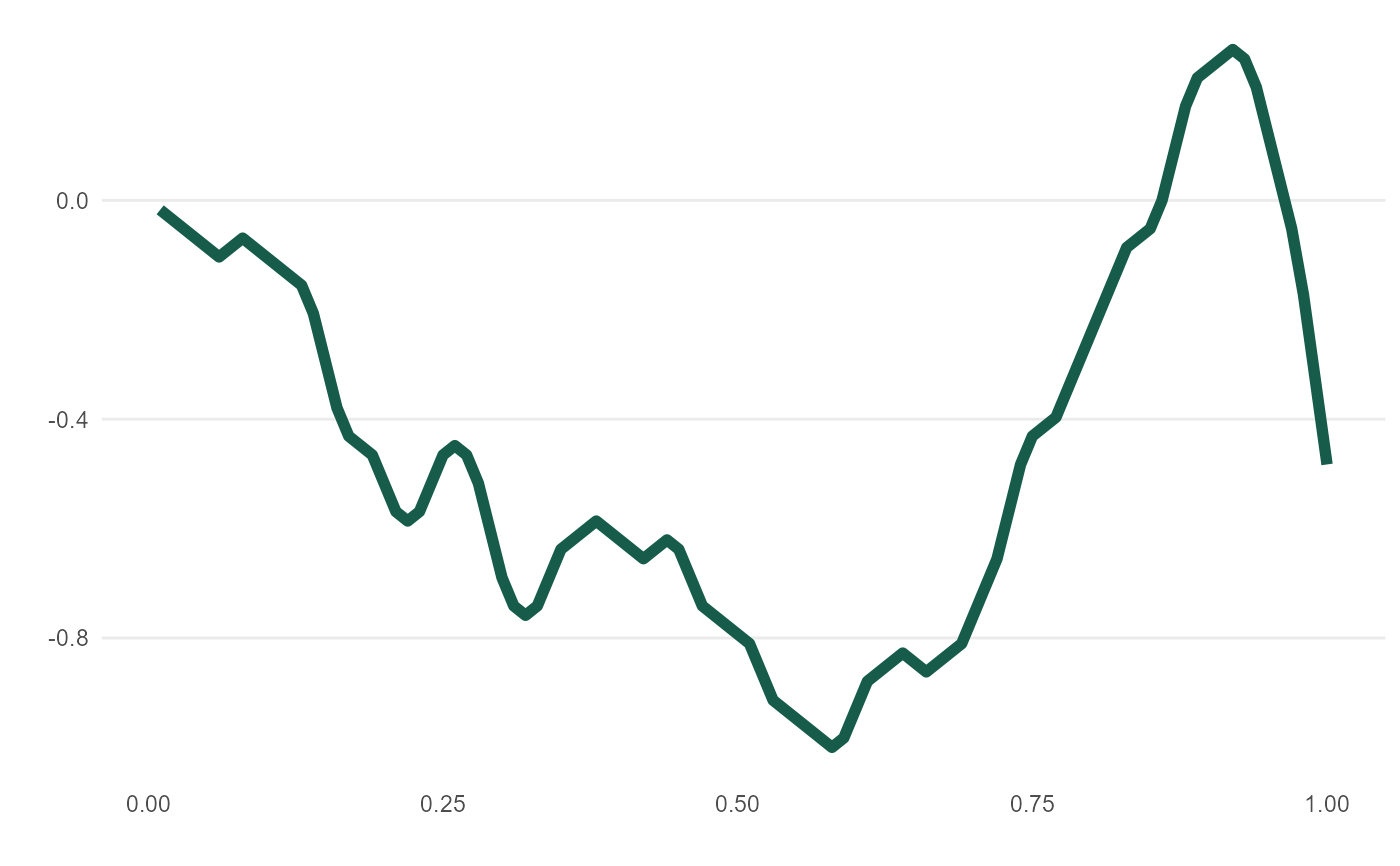Create simply line plot from x + y coordinates. Designed for meandr but can be used in other applications.

mplot(.df)

## Arguments

.df A data frame containing at least two numeric columns.

## Value

An object of class gg

## Examples

mplot(data.frame(x = 1:10, y = 1:10))mplot(meandr())